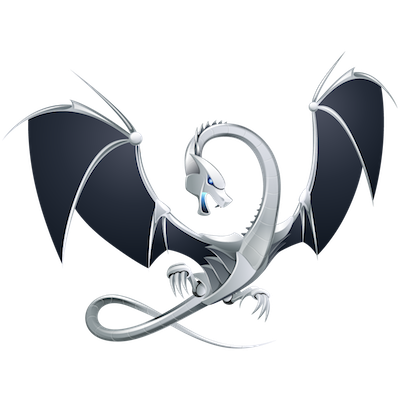# Luke Lau

Minm
Email
Git
GitHub

This site is HTTP2 ready 🚚💨blog :: [Post]$e^{i\pi}=-1$
$\left[\begin{array}{cc}1& {x}_{1}\\ {\int }_{0}^{\theta }f\left(x\right)dx& y\end{array}\right]$